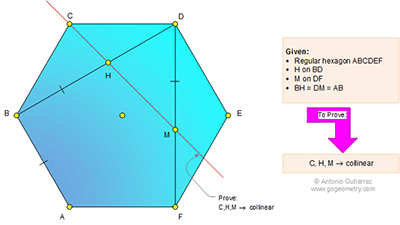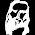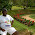## Thursday, April 2, 2015

### Problem 1106: Regular Hexagon, Congruence, Diagonal, Collinear Points

Geometry Problem. Post your solution in the comment box below.
Level: Mathematics Education, High School, Honors Geometry, College.

Click the diagram below to enlarge it.1.BCH and CDM are isosceles triangles
<(BCD)= 120 , <(CDM)= 90, <(CBD)= 30
<(BCH)= ½(180-30)= 75 => <(HCD)= 120-75= 45
<(DCM)= ½(180-90)= 45

<(HCD)=<(DCM)= 45 => C, H, M are collinear

2.Draw the segment CH: triangle BCH is isosceles and angle CBH = 30º (cause CB = CD and angle BCD is 120º) therefor the angle between BH and CH is (180-30)/2 = 75º .
Now let's find the angle between BH and HM: angle HDM = 60º cause triangle BDF is equilateral. Angle DMH = 45º because triangle DCM is isoceles and has angle CDM right. Therefore the angle between BH and HM = 75º.
This proofs that HM is paralel to HC but because both contain point H both must coincide.

3.Hey please don't aprove my last answer I used that CHM is a line to prove this exact fact.

4.Prove using section formula of vector.

2 vec(DH)
= 2 DH/DB vec(DB)
= 2 DH/DB (vec(DC) + vec(EF))
= 2 DH/DB (vec(DC) + 1/2 vec(DC) + 1/2 vec(DF))
= 3 DH/DB vec(DC) + DH/DB vec(DF)
= 3 DH/DB vec(DC) + DH/DB×DF/DM vec(DM)
= 3 DH/DB vec(DC) + DH/DM vec(DM)

Consider
3 DH/DB + DH/DM
= 3 (√3 - 1)/√3 + (√3 - 1)/1
= 2

Thus C, H, M are collinear.

5.Join CH and HM

Since BC = BH, < HCD = 45. Now since < CDM = 90, < MCD = 45
< HCD & < MCD can both be 45 only if C,H,M are collinear

Sumith Peiris
Moratuwa
Sri Lanka